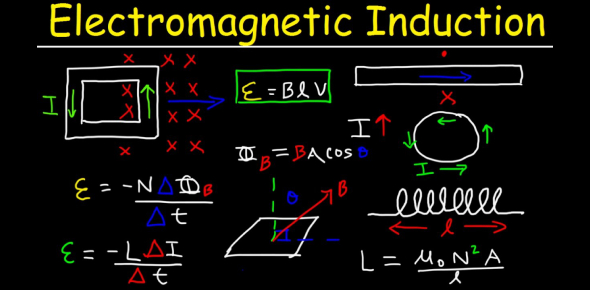# A Trivia Quiz On Electromagnetic Induction!

15 Questions | Total Attempts: 765Settings• 1.
The magnitude of EMF produced in a coil, when a magnet is inserted into it does not depend upon
• A.

Number of turns in the coil

• B.

Resentence of the coil

• C.

Magnetic moment of magnet

• D.

Speed of the magnet

• 2.
The ratio of primary and secondary turns in a transformer is 1: 200. If 220-volt a.c. is fed to primary, the voltage across secondary will be
• A.

44 V

• B.

220 V

• C.
• D.

40 k V

• 3.
The core of a transformer is laminated to
• A.

Reduce eddy current losses,

• B.

Increase magnetic flux

• C.

Increase strength of magnetic field,

• D.

Increase residual magnetism.

• 4.
The number of turns in the primary and the secondary of a transformer is 1000 and 3000 respectively. If 80-volt a.c. is applied to the primary coil of the transformer, then the potential difference per turn of the secondary coil would be
• A.

240 volt

• B.

2400 volt

• C.

0.24 volt

• D.

0.08 volt

• 5.
When a wire loop is related in a magnetic field the direction of included EMF change once in each.
• A.

¼ revolution

• B.

½ revolution

• C.

1 revolution

• D.

2 revolution

• 6.
A coil wire of a certain radius has 600 turns and a self-inductance of 108 mH. The self inductance of a second similar coil of 500 turns will be:
• A.

74 mH

• B.

75 mH

• C.

76 mH

• D.

77 mH

• 7.
A straight conductor of length 0.4 m is moved with a speed of 7 m/s perpendicular to a magnetic field of induction 0.9 webers per meter2. The included EMF. across the conductor is
• A.

25.2 V

• B.

5.04 V

• C.

2.52 V

• D.

1.26 V

• 8.
A long solenoid has 800 turns per meter. A current of 1.6 A flows through it. The magnetic induction at the end solenoid on its axis is
• A.

16×10-4 T

• B.

0.8 ×10-4 T

• C.

32×10-4  T

• D.

4×10-4 T

• 9.
A metallic square Loop ABCD is moving in its own plane with velocity v in a uniform magnetic field perpendicular to its plane as shown in Fig. 4 (CF) .15. An electric field is induced
• A.

• B.

In BC, but not in AD,

• C.

Neither in AD nor in BC

• D.

• 10.
Two circular coils can be arranged in any of the three situations shown in Fig. 4(CF).16. Their mutual inductance will be
• A.

Maximum in solutions (i)

• B.

Maximum in solutions (ii)

• C.

Maximum in situation (iii)

• D.

Same in all situation

• 11.
When a D.C motor operator at 200 V, its initial current is 5 A, but when it runs maximum speed, the current is only 3 A,  What is its back e.m.f.?
• A.

120 V

• B.

200 V

• C.

80 V

• D.

Zero

• 12.
A conducting square loop of side L and resistance R moves in it’s a uniform velocity v perpendicular to one of its sides. A magnetic induction B constant in time and space, pointing perpendicular and into the plane of the loop exists everywhere with half the loop outside the field, as shown in Fig, 4 (CF). 18. The induced e.m.f. is
• A.

BvR

• B.

VBL /R

• C.

VBL

• D.

BLv/2

• 13.
As shown in Fig. 4 (CF). 20, P, and Q are two co-axial conducting loops separated by some distance. When the switch S is closed, a clockwise current Ip flows in p ( as seen by E), and an induced current IQ1 flows in P (as seen by E ), and an induced current IQ1 flows in Q. The switch remains closed for a long time. When S is opened, a current IQ2 flows in Q. Then directions of IQ1 and IQ2 (as seen by E) are
• A.

Respectively clockwise and anticlockwise

• B.

Both clockwise

• C.

Both anticlockwise

• D.

Respectively anticlockwise and clockwise

• 14.
Two coils are placed close to each other. The mutual inductance of the pair of coils depends upon
• A.

The rates at which current are changing in the two coils

• B.

Relative position and orientation of the two coils

• C.

The material of the wires of the coils

• D.

The current in the two coils.

• 15.
The magnetic flux linked with the coil varies with time as  = 3 t2 +4t + 9. The magnitude of induced EMF at t = 2 s is
• A.

9 V

• B.

16 V

• C.

3 V

• D.

4 V

Related TopicsBack to top